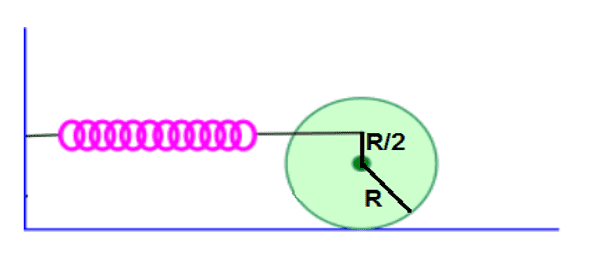# Where is my mistake? (Pure rolling and center of mass)

## Homework Statement

A cylinder with a mass of M and radius R is attached to a spring with a constant of k. The attachment always stays the same as described in the picture. The cylinder is moved to the right and the released, and it rolls without slipping (pure rolling). What will be the frequency of its movement? ICM=0.5MR^2 and the center of mass is at the middle.## Homework Equations

ma=F
Iα=Sum of torques.

## The Attempt at a Solution

I tried to the following.
first I think that the forces relevant are the forces applied by the spring and the friction from the floor. Then I wrote these equations:
MaCM=-kx+f where f is the friction.
ICMα=r1×F1+r2×F2=-0.5Rkx-Rf.

Then when I tried to say that a=αR (pure rolling) I get that f=0, which is not possible and gives a wrong answer.

Where is my mistake?

EDIT: From what I understand by reading the solution, the problem is that for some reason αR=a is incorrect but 1.5αR=a is correct. I have absolutely no idea why.

#### Attachments

Last edited:
•Delta2

BvU
Homework Helper
Hi,

One cause is that f appears with a + sign in the first and with a - sign in the second equation. Subtract them and you get f = 0.

Now for the physics:
What is the axis of rotation ? Where is the spring attached to the cylinder ?

haruspex
Homework Helper
Gold Member
2020 Award
The next step with such questions, after drawing the FBD, is to be very clear about which directions of displacement, acceleration, force, and rotation are positive.
Looks like you have right as positive for linear motion. Which way are taking as positive for rotation? Is that consistent with your a=αR equation? (Looks ok, just checking.)

Then when I tried to say that a=αR (pure rolling) I get that f=0,
Is that necessarily wrong?

One cause is that f appears with a + sign in the first and with a - sign in the second equation. Subtract them and you get f = 0.
The signs look ok to me.

Last edited:
•BvU
The next step with such questions, after drawing the FBD, is to be very clear about which directions of displacement, acceleration, force, and rotation are positive.
Looks like you have right as positive for linear motion. Which way are taking as positive for rotation? Is that consistent with your a=αR equation? (Looks ok, just checking.)

Is that necessarily wrong?

The signs look ok to me.
I thought it was consistent. First of all I can't understand how is it possible for pure rolling to occur without friction. Also, if we assume that those equation are correct I get ω=√(k/m) while the answer is ω=√(3k/2m) so obviously something is wrong.

I'll try to translate the question again.

A cylinder with a mass of M and radius R is attached to a spring with a constant of K. The attachment always stays the same as described in the picture (the distance between the center of the cylinder and the attachment is always R/2). We move the center of the cylinder to the right and release it. The cylinder rolls without slipping (pure rolling). What is the frequency of the motion? ICM=0.5MR^2, the center of mass is at the center of the cylinder.

From what I understand by reading the solution, the problem is that for some reason αR=a is incorrect but 1.5αR=a is correct. I have absolutely no idea why.

haruspex
Homework Helper
Gold Member
2020 Award
I can't understand how is it possible for pure rolling to occur without friction
Rolling just means there is no relative motion between the points in contact.
Think of just the wall, spring and cylinder, no floor, no gravity. In fact, replace the spring by some arbitrary constant force pushing or pulling on the cylinder at some point.
If the force is applied at the centre of the cylinder, it won't rotate at all. The further away from the centre, the greater the rotation, but the linear acceleration stays the same.
At some point, the rotation is so great that parts of the cylinder, on the side away from where the force is applied, move the opposite way to the cylinder's centre.
At exactly the right distance (R/2), the point of the cylinder furthest from the applied force has no linear acceleration in the force direction.

Last edited:
haruspex
Homework Helper
Gold Member
2020 Award

From what I understand by reading the solution, the problem is that for some reason αR=a is incorrect but 1.5αR=a is correct. I have absolutely no idea why.
That's not the answer I get. Please post the whole solution if you can.

Delta2
Homework Helper
Gold Member
I have problem understanding how the attachment of the spring to the cylinder remains always at that point and at that angle, while the cylinder moves and rotates.

There must be some sort of patented mechanism which of course is not shown, right?

haruspex
•